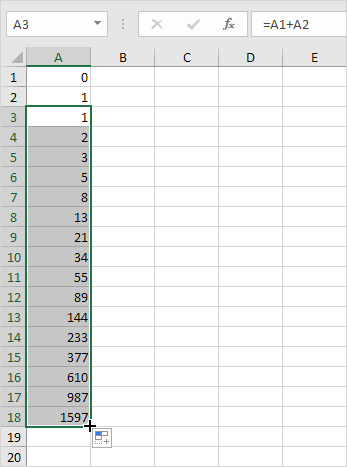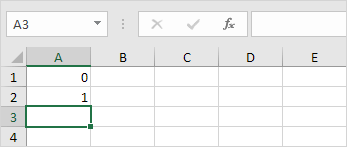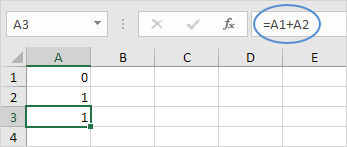# How to create Fibonacci Sequence in Excel

Jan 31, 2020 • edited Feb 01, 2020Fibonacci numbers form a sequence. After two starting numbers, the next number in the sequence is the sum of the two preceding numbers. The Fibonacci 10 numbers in the Fibonacci sequence are: 0, 1, 2, 3, 5, 8, 13, 21, 44, 65. Here are the steps to create a Fibonacci sequence in Excel:

It's easy to create all sorts of sequences in Excel.

1. The first two numbers in the Fibonacci sequence are 0 and 1.2. Each subsequent number can be found by adding up the two previous numbers.3. Click on the lower right corner of cell A3 and drag it down.

Result. The Fibonacci Sequence in Excel.#How To#Tutorial#Range#Fibonacci Sequence

How To Create A Custom List In Excel

How to Use AutoFill in Excel